expression in x, assume the function so that Subtracting equation (4.2) from equation (4.3) gives and dividing both sides of the equation by x, we have The desired formula is obtained by taking the limit of both sides as x approaches zero so that"> General FormulaCustom SearchGENERAL FORMULA To obtain a formula for the derivative of any expression in x, assume the function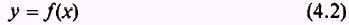so that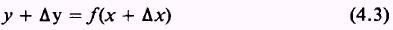Subtracting equation (4.2) from equation (4.3) givesand dividing both sides of the equation byx, we have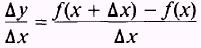The desired formula is obtained by taking the limit of both sides asx approaches zero so that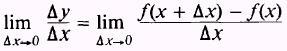or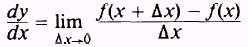NOTE: The notationis not to be considered as a fraction in which dy is the numerator and dx is the denominator. The expression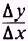is a fraction withy as its numerator andx as its denominator. Whereas,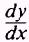is a symbol representing the limit approached by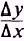asx approaches zero. EXAMPLES OF DIFFERENTIATION In this last section of the chapter, we will use several examples of differentiation to obtain a firm understanding of the general formula. EXAMPLE: Find the derivative,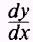-, for the functiondetermine the slopes of the tangent lines to the curve at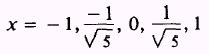and draw the graph of the function. SOLUTION: Finding the derivative by formula, we haveandExpand equation (1), then subtract equation (2) from equation (1) , and simplify to obtain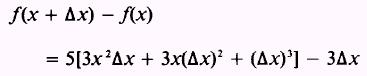Dividing both sides by Ax, we haveTake the limit of both sides as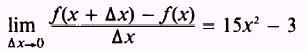Then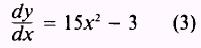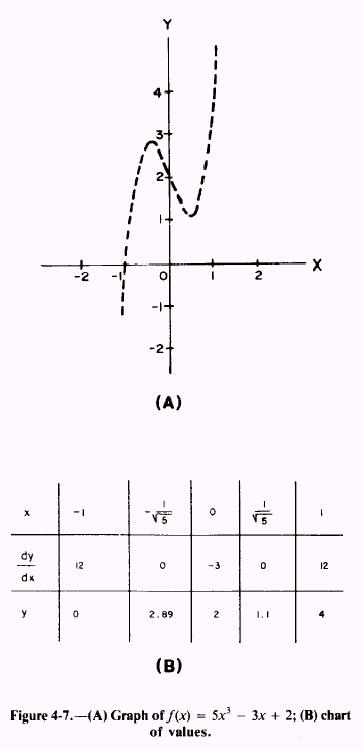The slopes of the tangent lines to the curve at the points given, using this derivative, are shown in figure 4-7, view B. Thus we have a new method of graphing an equation. By substituting different values of x in equation (3), we can find the slope of the tangent line to the curve at the point corresponding to the value of x. The graph of the curve is shown in figure 4-7, view A. EXAMPLE: Differentiate the function; that is, find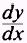of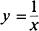and then find the slope of the tangent line to the curve at SOLUTION: Apply the formula for the derivative, and simplify as follows:Now take the limit of both sides as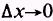so that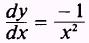To find the slope of the tangent line to the curve at the point where x has the value 2, substitute 2 for x in the expression for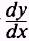.EXAMPLE: Find the slope of the tangent line to the curveAt x=3 SOLUTION: We need to find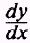, which is the slope of the tangent line at a given point. Apply the formula for the derivative as follows: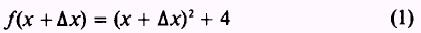and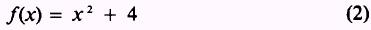Expand equation (1) so that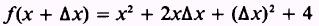Then subtract equation (2) from equation (1):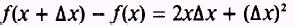Now, divide both sides by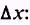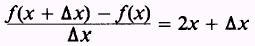Then take the limit of both sides asSubstitute 3 for x in the expression for the derivative to find the slope of the tangent line at x=3 so that slope = 6 In this last example we will set the derivative of the function, f (x), equal to zero and determine the values of the independent variable that will make the derivative equal to zero to find a maximum or minimum point on the curve. By maximum or minimum of a curve, we mean the point or points through which the slope of the tangent line to the curve changes from positive to negative or from negative to positive. NOTE: When the derivative of a function is set equal to zero, that does not mean in all cases we will have found a maximum or minimum point on the curve. A complete discussion of maxima or minima may be found in most calculus texts. To set the derivative equal to zero, we will require that the following conditions be met: 1. We have a maximum or minimum point. 2. The derivative exists. 3. We are dealing with an interior point on the curve. When these conditions are met, the derivative of the function will be equal to zero. EXAMPLE: Find the derivative of the functionset the derivative equal to zero, and find the points of maximum and minimum on the curve. Then verify this by drawing the graph of the curve. SOLUTION: Apply the formula for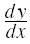as follows:and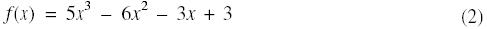Expand equation (1) and subtract equation (2), obtainingNow, divide both sides by, and take the limit as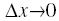so thatSet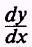equal to zero; thus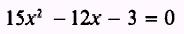Then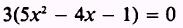andSet each factor equal to zero and find the points of maximum or minimum; that is,and x=1 The graph of the function is shown in figure 4-8.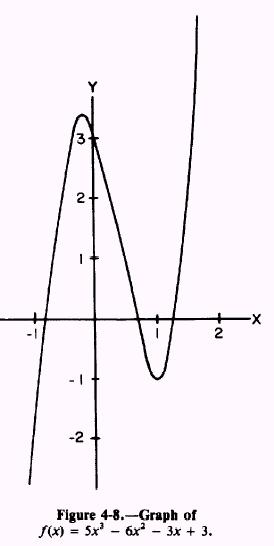Integrated Publishing, Inc. - A (SDVOSB) Service Disabled Veteran Owned Small Business